# Texas Go Math Grade 5 Lesson 17.1 Answer Key Income and Payroll Taxes

Refer to our Texas Go Math Grade 5 Answer Key Pdf to score good marks in the exams. Test yourself by practicing the problems from Texas Go Math Grade 5 Lesson 17.1 Answer Key Income and Payroll Taxes.

## Texas Go Math Grade 5 Lesson 17.1 Answer Key Income and Payroll Taxes

Calculate pay after taxes.

Mia earns $600 per week. When she gets her paycheck, it includes a pay stub that shows total earnings and payroll tax. Find Mia’s pay after taxes.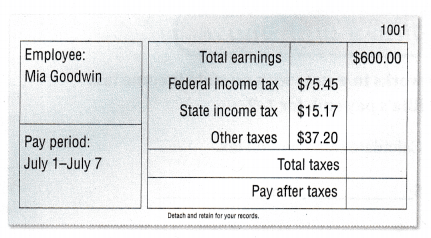Mia earns$600 per week. When she gets her paycheck, it includes a pay stub that shows total earnings and payroll tax. Find Mia’s pay after taxes.

Circle the taxes taken out of Mia’s paycheck.

Add the taxes to calculate the payroll tax.
$_______ +$_____ + $____ =$______

Subtract the payroll tax from her total earnings.

$600 –$____ = ______
So, Mia’s pay after taxes is $____. Answer: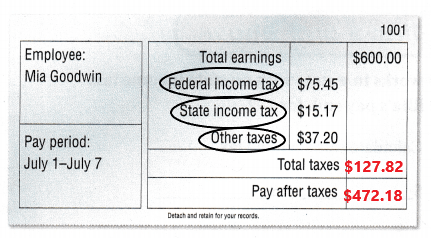Add the taxes to calculate the payroll tax.$75.45 + $15.17 +$37.20 = $127.82 Subtract the payroll tax from her total earnings.$600 – $127.82 =$472.18
So, Mia’s pay after taxes is $472.18. Explanation: Mia earns$600 per week. When she gets her paycheck, it includes a pay stub that shows total earnings and payroll tax. In the above table we can observe different types of taxes and total earning.
To calculate the payroll tax first we have to add the taxes of federal income tax, state income tax, other taxes. Add $75.45 with$15.17 and 37.20 then the sum is $127.82. The payroll tax is$127.82.
Next we have to calculate Mia’s pay after taxes. Subtract the payroll tax from her total earnings. The total earnings are $600. Subtract$127.82 from $600 then the difference is$472.18. Mia’s pay after tax is $472.18. Example Rob earns$500 per week. He has payroll tax taken out of his earnings. Unfortunately, Rob spilled kale juice on his pay stub, covering up some of the values. He knows the Other taxes are $10. How much state income tax was withheld from Rob’s earnings?Find the payroll tax withheld from Rob’s total earnings.$ ____ – $____ =$______

Add the federal income tax and other taxes.
$____ +$____ = $______ Subtract the total of federal income tax and other taxes from the payroll tax to find the state income tax.$ ____ – $____ or$______
So, Rob’s state income tax is $_____ Answer: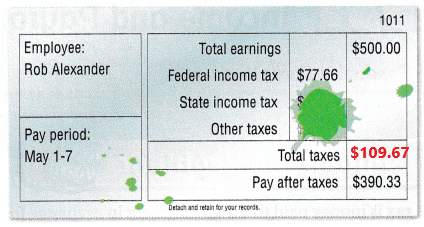Find the payroll tax withheld from Rob’s total earnings.$500 – $390.33 =$109.67
Add the federal income tax and other taxes.
$77.66 +$10 = $87.66 Subtract the total of federal income tax and other taxes from the payroll tax to find the state income tax.$109.67 – $87.66 =$22.01
So, Rob’s state income tax is $22.01. Explanation: Rob earns$500 per week. He has payroll tax taken out of his earnings. Unfortunately, Rob spilled kale juice on his pay stub, covering up some of the values. He knows the Other taxes are $10. In the above table we can observe total earnings, federal income tax and pay after taxes. First we have to subtract pay after taxes from total earnings to find the payroll tax withheld from Rob’s total earnings. Subtract$390.33 from $500 then the difference is$109.67. The payroll tax is $109.67. Second we have to add the federal income tax and other taxes. Add$77.66 with $10 then the sum is$87.66.
Third we have to Subtract the total of federal income tax and other taxes from the payroll tax to find the state income tax. Subtract $87.66 from$109.67 then the difference is $22.01. So, Rob’s state income tax is$22.01.

Share and Show

Rita works In a state with no state income tax. Use Rita’s pay stub for 1-2.

Question 1.
Calculate Rita’s payroll tax.
$______ +$________
= $________ Answer: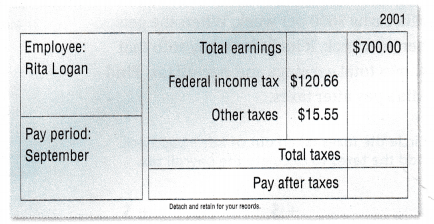Answer: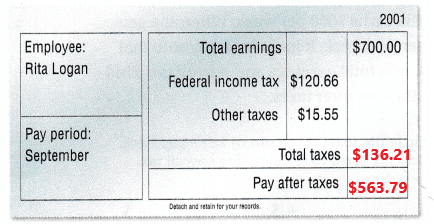Rita’s payroll tax =$120.66 + $15.55 =$136.21.
Explanation:
Rita works In a state with no state income tax. In the above image we can observe Total earnings, Federal income tax and other taxes. Here we have to find out the payroll tax. Rita’s payroll tax is calculated by adding federal income tax and other taxes. Add $120.66 with$15.55 then the sum is $136.21. Rita’s payroll tax is$136.21.

Question 2.
Calculate Rita’s pay after taxes.
$____ –$______ =$_____ Answer: Rita’s pay after taxes$700 – $136.21 =$563.79.
Explanation:
Rita works In a state with no state income tax. In the above image we can observe Total earnings, Federal income tax and other taxes. Here we have to find out the Rita’s pay after taxes. From the above question 1 we have Rita’s payroll tax is $136.21. Subtract Rita’s payroll tax from total earnings then we get the Rita’s pay after taxes. Subtract$136.21 from $700 then the difference is$563.79. Rita’s pay after taxes is $563.79. Problem Solving Question 3. Write Math Explain how you can calculate payroll tax if you know total earnings and pay after taxes. Answer: Consider total earnings is$700 and pay after taxes is $563.$700 – $563 =$137
The payroll tax is $137. Explanation: If we know total earnings and pay after taxes then we can easily calculate the payroll tax. By subtracting pay after taxes from total earnings then the difference is nothing but the payroll tax. Consider total earnings is$700 and pay after taxes is $563. Subtract$563 from $700 then the difference is$137. The payroll tax is $137. Problem Solving Question 4. Leticia pays federal income tax of$18 for every $100 she earns. How much will she pay as federal income tax if she earns$500? Explain.
Federal income tax of $18 for every$100 she earns.
$100 =$18
$500 =$?
$500 x$18 = $100 x ? ($9000)/($100) =$90
Leticia has to pay $90 as federal income tax, if she earns$500.
Explanation:
Leticia pays federal income tax of $18 for every$100 she earns. We have to calculate the federal income tax she has to pay if she earns $500. First multiply$500 with $18 then the product is$9000. Divide $9000 by$100 then the quotient is $90. Leticia has to pay$90 as federal income tax, if she earns $500. Question 5. Michelle works for 33 hours this week. She earns$10 per hour. Her pay after taxes is $277.11. How much does she pay in payroll tax? Answer: 1hour =$10
33hours = ?
33 hours x $10 = 1 hour x ?$330/1 = $330 Michelle earns$330.
$330 –$277.11 = $52.89 Michelle pays the payroll tax$52.89.
Explanation:
Michelle works for 33 hours this week. She earns $10 per hour. First multiply 33 hours with$10 then the product is $330. Michelle earns$330. Her pay after taxes is $277.11. To calculate the payroll tax we have to subtract pay after taxes from total earnings. Subtract$277.11 from $330 then the difference is$52.89. Michelle pays the payroll tax $52.89. Question 6. H.O.T. Multi-Step Oliver lives in a state with no state income tax. He pays$150 in payroll tax each week. If the federal income tax is 0.75 of the payroll tax, how much are the other taxes?Payroll tax each week is $150. Federal income tax = 0.75 x$150 = $112.5 Other taxes = ? Payroll tax = Federal income tax + other taxes$150 = $112.5 + other taxes Other taxes =$150 – $112.5 =$37.5
Explanation:
Oliver lives in a state with no state income tax. He pays $150 in payroll tax each week. The federal income tax is 0.75 of the payroll tax. Multiply 0.75 with$150 the product is $112.5. Federal income tax is$112.5. Here we have to calculate the other taxes. Subtract Federal income tax $112.5 from payroll tax$150 then the difference is $37.5. The other taxes are$37.5.

Question 7.
H.O.T. Multi-Step The pay stub shows the payroll tax withheld from Maeve’s paycheck. If Maeve gets paid twice a month, how much does she pay in payroll tax in one month? Explain.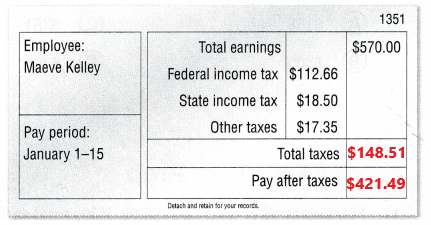The pay roll tax for 15 days.
$112.66 +$18.50 + $17.35 =$148.51
The pay roll tax for one month.
$148.51 +$148.51 = $297.02 The pay roll tax for one month is 297.02 The pay after tax for 15 days.$570 – $148.51 =$421.49
Pay after taxes for one month.
$1140 -$297.02 = $842.98 Explanation: The pay stub shows the payroll tax withheld from Maeve’s paycheck. Maeve gets paid twice a month, We have to calculate the pay roll tax for one month. In the above table we can observe total earning, Federal income tax, state income tax and other taxes. First we have to calculate the pay roll tax for 15 days. Add Federal income tax with state income tax and other taxes. Add$112.66 with $18.50 and$17.35 then the sum is $148.51. The pay roll tax for 15 days is$148.51.
Second we have to calculate the pay roll tax for one month. So, add $148.51 with$148.51 then the sum is $297.02. The pay roll tax for one month is$297.02.
The pay after tax for 15 days is calculated by subtracting payroll tax from total earnings. Subtract $148.51 from$570 then the difference is $421.49. The pay after tax for 15 days is$421.49.
The pay after tax for one month is calculated by subtracting payroll tax from total earnings. Subtract $297.02 from$1140 then the difference is $842.98. Pay after taxes for one month is$842.98.

Question 8.
Arman works in a state with no state income tax. He earns $625 each week. He pays$38.45 in other taxes. His pay after taxes is $499.61. How much federal income tax does he pay? (A)$38.45
(B) $76.90 (C)$86.94
(D) $88.72 Answer:Total earning is equal to$625 for week.
His pay after taxes is $499.61. Total taxes is$625 – $499.61 =$125.39.
Other taxes is $38.45. Federal income tax is equal to$125.39 – $38.45 =$86.94.
So, option C is correct.
Explanation:
Arman works in a state with no state income tax. He earns $625 each week. He pays$38.45 in other taxes. His pay after taxes is $499.61. Here we have to calculate the federal income tax he has to pay. The payroll is calculated by subtracting pay after taxes from total earnings. Subtract$499.61 from $625 then the difference is$125.39. Arman payroll tax is $125.39. Federal income tax is calculated by subtracting other taxes from payroll tax. The payroll tax is nothing but total taxes. Subtract$38.45 from $125.39 then the difference is$86.94. The Federal income tax is $86.94. So option C is correct. Question 9. Communicate Miranda earns$450.75 at her part-time job. She pays $98.12 in federal income tax and$12.00 in other taxes. How can you determine Miranda’s pay after taxes?
(A) Subtract the sum of the taxes from the total earnings.
(B) Subtract the amount earned from the sum of the taxes.
(D) Subtract only the federal income tax from the total earnings.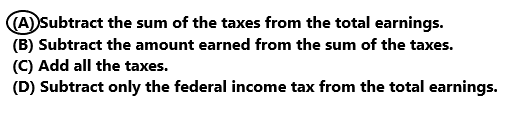Option A is correct.
Explanation:
Miranda earns $450.75 at her part-time job. She pays$98.12 in federal income tax and $12.00 in other taxes. To determine Miranda’s pay after taxes we have to subtract the sum of the taxes from the total earnings. So, option A is correct. Question 10. Multi-Step Joe has two part-time jobs. At his first job, he earned$200 and paid $42 in payroll tax. At his second job he earned$175 and paid $24 in payroll tax. What is his pay after taxes? (A)$158
(B) $309 (C)$351
(D) $303 Answer: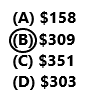At his first job, he earned$200 and paid $42 in payroll tax.$200 – $42 =$158
At his second job he earned $175 and paid$24 in payroll tax.
$175 -$24 = $151 Joe pay after taxes are$158 + $151 =$309
So, option B is correct.
Explanation:
Joe has two part-time jobs. At his first job, he earned $200 and paid$42 in payroll tax. At his second job he earned $175 and paid$24 in payroll tax. Here we have to calculate the pay after taxes.
At his first job, he earned $200 and paid$42 in payroll tax. Subtract payroll tax from total earnings then we get pay after tax. Subtract $42 from$200 then the difference is $158. At his first job the pay after tax is$158.
At his second job, he earned $175 and paid$24 in payroll tax. Subtract payroll tax from total earnings then we get pay after tax. Subtract $24 from$175 then the difference is $151. At his second job the pay after tax is$151.
Add first pay after tax$158 with second pay after tax$151 then the sum is $309. Joe’s pay after taxes are$309. So option B is correct.

Test Prep

Question 11.
Mark’s pay after taxes is $855.08. If his total earnings are$1,100, how much does Mark pay in payroll tax?
(A) $1,100 (B)$144.02
(C) $244.92 (D)$855.08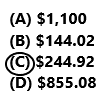Mark’s total earnings are $1,100. Mark’s pay after taxes is$855.08.
$1,100 –$855.08 = $244.92 Mark pay in payroll tax is$244.92.
So option C is correct.
Explanation:
Mark’s pay after taxes is $855.08. His total earnings are$1,100. Subtract pay after taxes from total earnings then the difference is equal to the payroll tax. Subtract $855.08 from$1,100 the difference is $244.92. Mark pay in payroll tax is$244.92. So option C is correct.

### Texas Go Math Grade 5 Lesson 17.1 Homework and Practice Answer Key

Question 1.
Write an equation to show how to calculate the total amount Sam pays in payroll tax.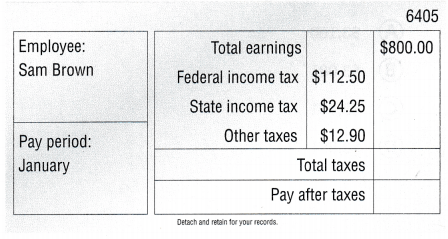The payroll tax is $112.50 +$24.25 + $12.90 =$149.65.
The total tax is $149.65. Explanation: In the above table we have a data of total earnings, Federal income tax, state income tax and other taxes. To calculate the total amount Sam pays in payroll tax, we need to add the Federal income tax, state income tax and other taxes. Add$112.50 with $24.25 and$12.90 then the sum is $149.65. Sam payroll tax is$14.65.

Question 2.
Use your solution in Exercise 1 to write an equation to calculate Sam’s pay after taxes if his total earnings are $800. Answer: Sam’s pay after taxes is$800 – $149.65 =$650.35.
Explanation:
Use the solution in Exercise 1 to write an equation to calculate Sam’s pay after taxes. Sam’s total earnings are $800. To get Sam’s pay after taxes we have to subtract the payroll tax from total earnings of Sam’s. Subtract$149.65 from $800 then the difference is$650.35. Sam’s pay after taxes is $650.35. Question 3. Explain how you can calculate total earnings if you know the payroll tax and the pay after taxes. Answer: Total earnings = Payroll tax + Pay after taxes Total earnings =$149.65 + $650.35 Total earnings =$800
Explanation:
We can calculate the total earnings if we know the payroll tax and pay after taxes. Total earnings is calculated by adding payroll tax with pay after taxes. Add $149.65 with 650.35 then the sum is$800. The total earnings are $800. Problem Solving Question 4. The pay stub at right shows Dana’s earnings and taxes this week. Darla says her pay after taxes will be an amount greater than$600. Explain how you can use estimation to decide if Darla is correct.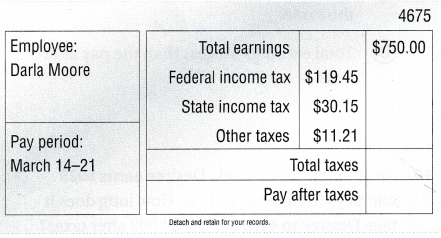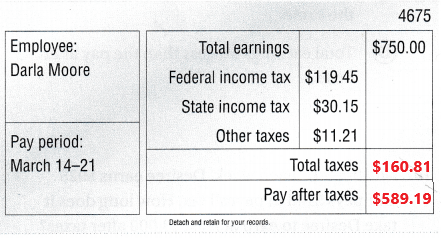Explanation:

Question 5.
Adolfo earned $650 last week. He paid$97.50 in federal income tax. He paid one third that amount for state income tax and other taxes combined. What was Adolfo’s pay after taxes? Explain how you found your answer.
Total earning is $650. He paid Federal income tax$97.50.
He paid one third that amount for state income tax and other taxes combined.
(1/3) x $650 =$216.66
Payroll tax = $97.50 +$216.66 = $314.16 Adolfo’s pay after taxes = Total earning – Payroll tax Adolfo’s pay after taxes =$650 – $314.16 Adolfo’s pay after taxes =$335.84
Explanation:
Adolfo earned $650 last week. He paid$97.50 in federal income tax. He paid one third that amount for state income tax and other taxes combined. Multiply (1/3) with $650 then the product is$314.16. Adolfo’s pay after taxes is calculated by subtracting payroll tax from total earnings. Subtract $314.16 from$650 the difference is $335.84. Adolfo’s pay after taxes is$335.84.

Lesson Check

Texas Test Prep

Question 6.
Martina earns $4,000 per month. Each month, she pays$680 in federal income tax, $160 in state income tax, and$79 in other taxes. What is Martina’s pay after taxes each month?
(A) $3,160 (B)$3,081
(C) $3,761 (D)$2,981Martina pays $680 in federal income tax,$160 in state income tax, and $79 in other taxes.$680 + $160 +$79 = $919 Martina’s pay after taxes each month is$4,000 – $919 = 3,081. So, option B is correct. Explanation: Martina earns$4,000 per month. Each month, she pays $680 in federal income tax,$160 in state income tax, and $79 in other taxes. To calculate the payroll tax we need to add the Federal income tax with State income tax and other taxes. Add$680 with $160 and$79 then the sum is $919. Martina’s payroll tax is$919. Subtract payroll tax from total earnings then we get the Martina’s pay after taxes. Subtract $919 from$4,000 then the difference is $3,081. Martina’s pay after taxes each month is$3,081. So, option B is correct.

Question 7.
Lacey earns $680 each week. She pays$91.80 in federal income tax. The other taxes are $17.00. Her pay after taxes is$547.40. How much does Lacey pay in state income tax?
(A) $74.80 (B)$38.60
(C) $115.60 (D)$23.80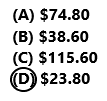Lacey earns $680 each week. Her pay after taxes is$547.40.
$680 –$547.40 = $132.6 Total payroll tax is$132.6.
She pays $91.80 in federal income tax. The other taxes are$17.00.
Total payroll tax = Federal income tax + State income tax + other taxes
$132.6 =$91.80 + State income tax + $17.00 State income tax =$132.6 – $91.80 –$17.00
State income tax = $23.8. So, option D is correct. Explanation: Lacey earns$680 each week. She pays $91.80 in federal income tax. The other taxes are$17.00. Her pay after taxes is $547.40. We have to calculate the State income tax. Subtract Federal income tax and other taxes from total payroll then the difference is equal to the state income tax. Subtract$91.80 and $17.00 from$132.6 then the difference is $23.8. Lacey’s state income tax is$23.8. So option D is correct.

Question 8.
Which statement about total earnings and pay after taxes is correct, if the payroll tax is withheld?
(A) Pay after taxes is greater than total earnings.
(B) Pay after taxes is less than total earnings.
(C) Total earnings and pay after taxes are the same.
(D) Total earnings are less than the pay after taxes.Option B is correct.
Explanation:
Option B pay after taxes is less than total earnings is correct.

Question 9.
Each week, Kelly pays $68.45 in federal income tax,$22.81 in state income tax, and $18.75 in other taxes. Which is the best estimate for the payroll tax Kelly pays each month? (A)$440
(B) $110 (C)$280
(D) $360 Answer: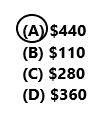Each week, Kelly pays$68.45 in federal income tax, $22.81 in state income tax, and$18.75 in other taxes.
$68.45 +$22.81 + $18.75 =$110.01
For one week Kelly pays payroll tax is $110. For one month Kelly pays payroll tax$440.
Option A is correct.
Explanation:
Each week, Kelly pays $68.45 in federal income tax,$22.81 in state income tax, and $18.75 in other taxes. The pay roll tax for one week is calculated by adding Federal income tax with state income tax and other taxes. Add$68.45 with $22.81 and$18.75 then the sum is $110.01. We have 4 weeks in a month. So multiply 4 with 110 then the product is$440. The best estimation for payroll tax Kelly pays each month is $440. So option A is correct. Question 10. Multi-Step Each week, Desiree earns$925 and pays $148 in payroll tax. How long does it take Desiree to earn at least$3,000 after taxes?
(A) 4 weeks
(B) 3 weeks
(C) 5 weeks
(D) 7 weeks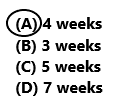For one week, Desiree earns $925 and pays$148 in payroll tax.
$925 –$148 = $777 Desiree to earn at least$3,000 after taxes.
$777 x 4 weeks =$3,108
So, Option A is correct.
Explanation:
Multi-Step Each week, Desiree earns $925 and pays$148 in payroll tax. By subtracting the payroll tax from total earnings we get the pay after tax. Subtract $148 from$925 the difference is $777. Desiree to earn at least$3,000 after taxes. Multiply $777 with 4 weeks then the product is$3,108.  So option A is correct.

Question 11.
Multi-Step Brian works 24 hours this week. His earns $9.25 per hour. He pays$37.30 in payroll tax. He deposits half of his pay after taxes in his savings account. He deposits the other half of his pay after taxes in his checking account. How much money is deposited into each account?
(A) $184.70 (B)$129.65
(C) $92.35 (D)$46.55Multi-Step Brian works 24 hours this week. His earns $9.25 per hour. 1 hour =$9.25
24 x $9.25 =$222
Total earnings is $222. He pays$37.30 in payroll tax.
$222 –$37.30 = $184.7 The pay after taxes is$184.7.
($184.7)/2 =$92.35
He deposited $92.35 in each account. So, option C is correct. Explanation: Multi-Step Brian works 24 hours this week. His earns$9.25 per hour. Multiply 24 hours with $9.25 then the product is$222. Total earning is $222. He pays$37.30 in payroll tax. By subtracting payroll tax from total earnings we get the pay after taxes. Subtract $37.30 from$222 then the difference is $184.7. The pay after taxes is$184.7. He deposits half of his pay after taxes in his savings account. He deposits the other half of his pay after taxes in his checking account. Divided $184.7 by 2 then the quotient is$92.35. He deposited \$92.35 in each account. So option C is correct.